Ex 11.2

Chapter 11 Class 10 Constructions [Deleted]
Serial order wise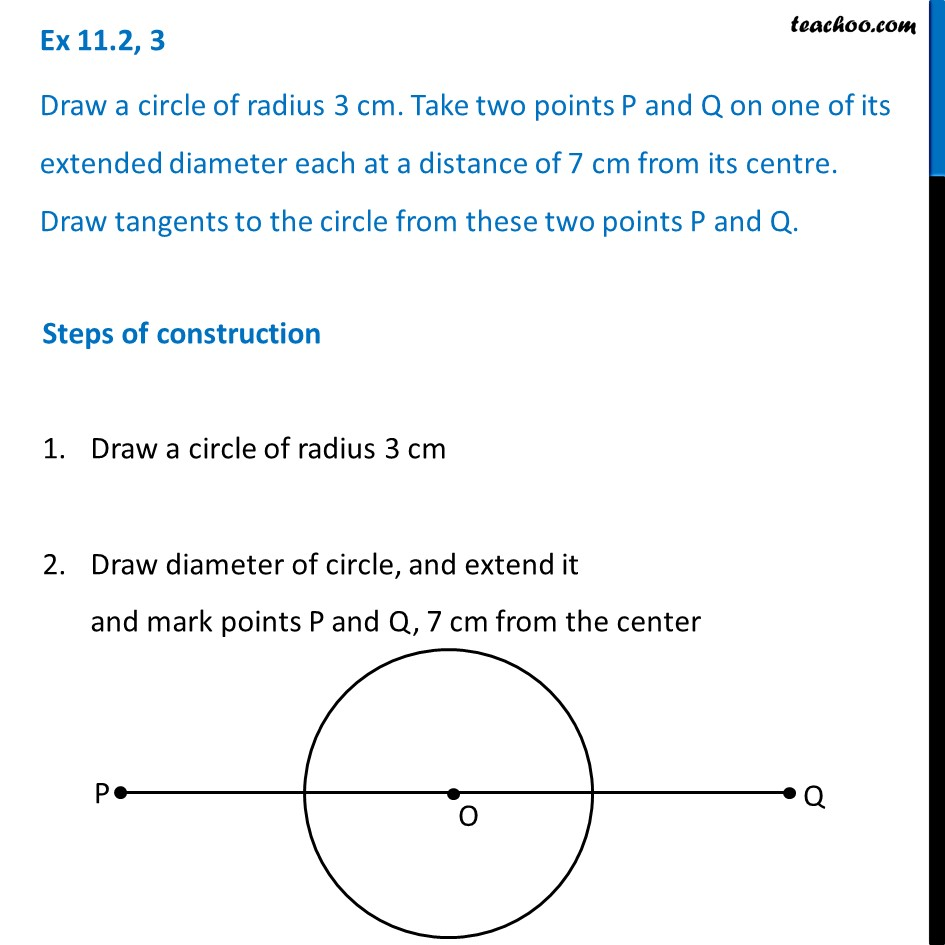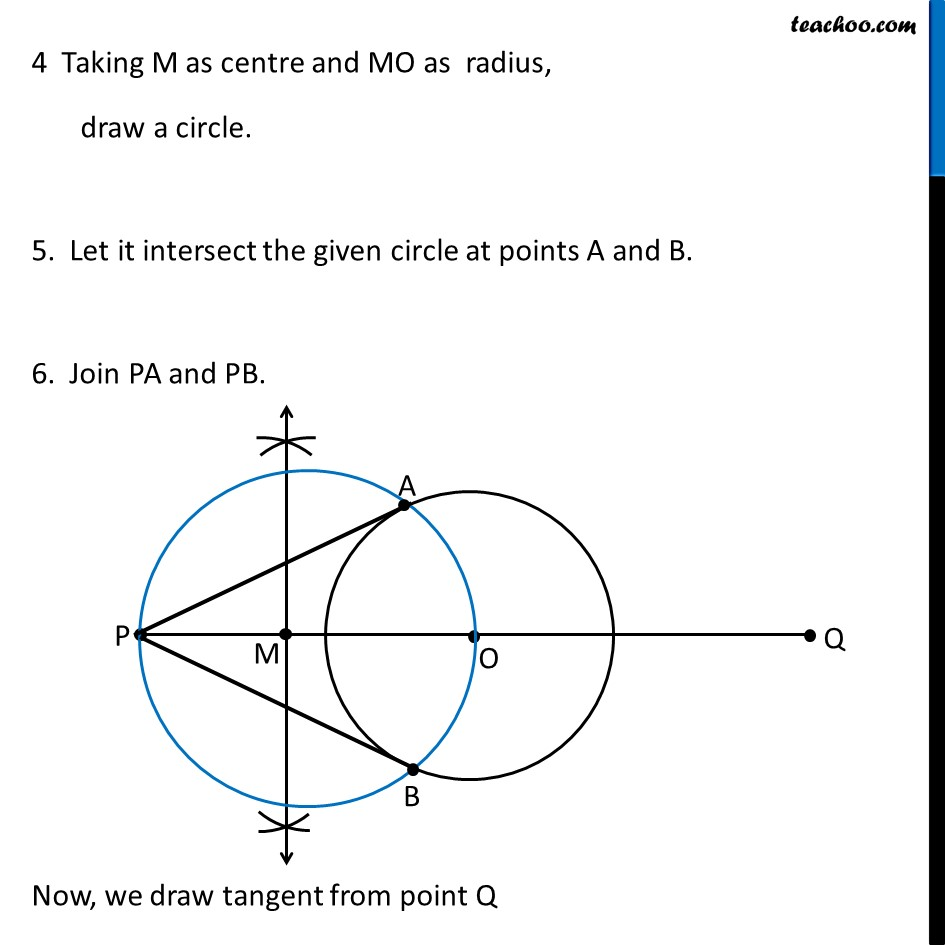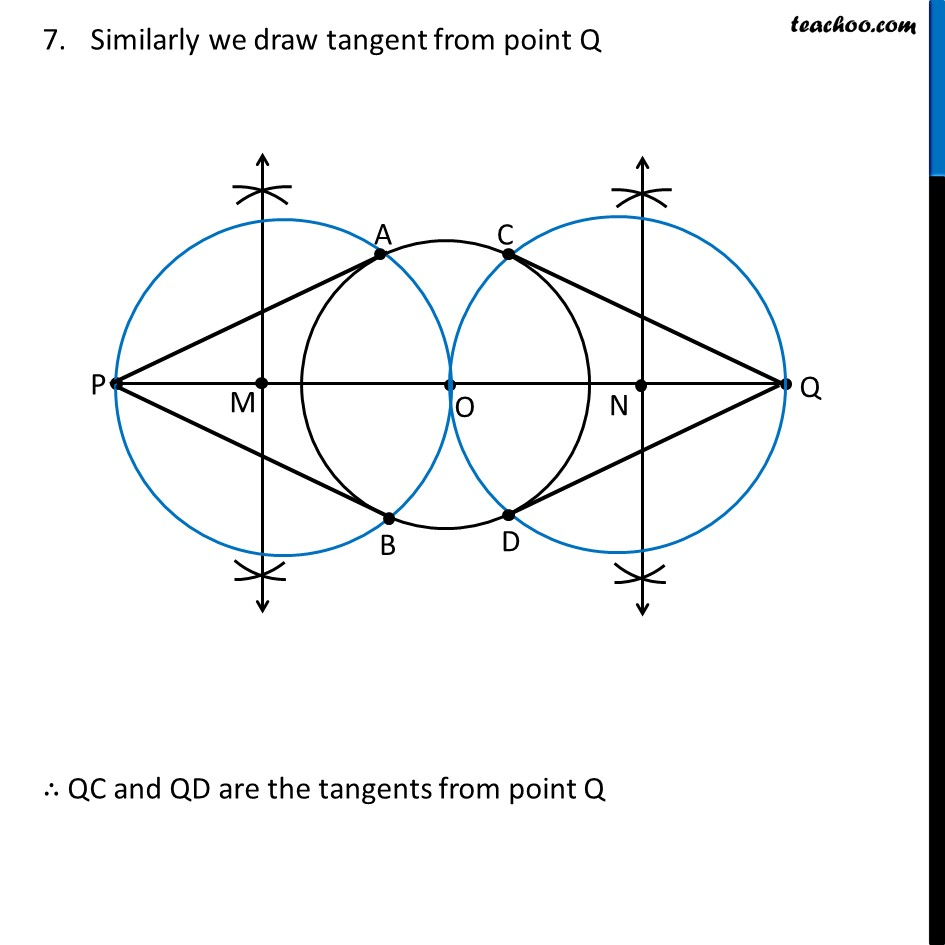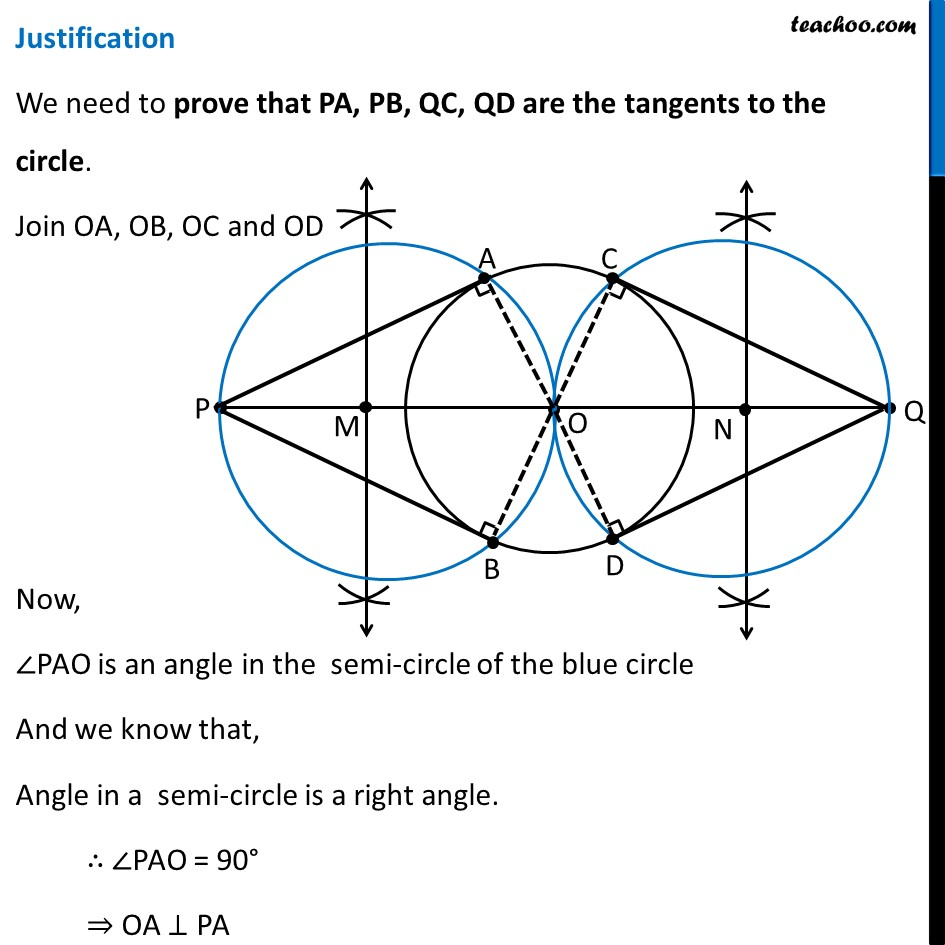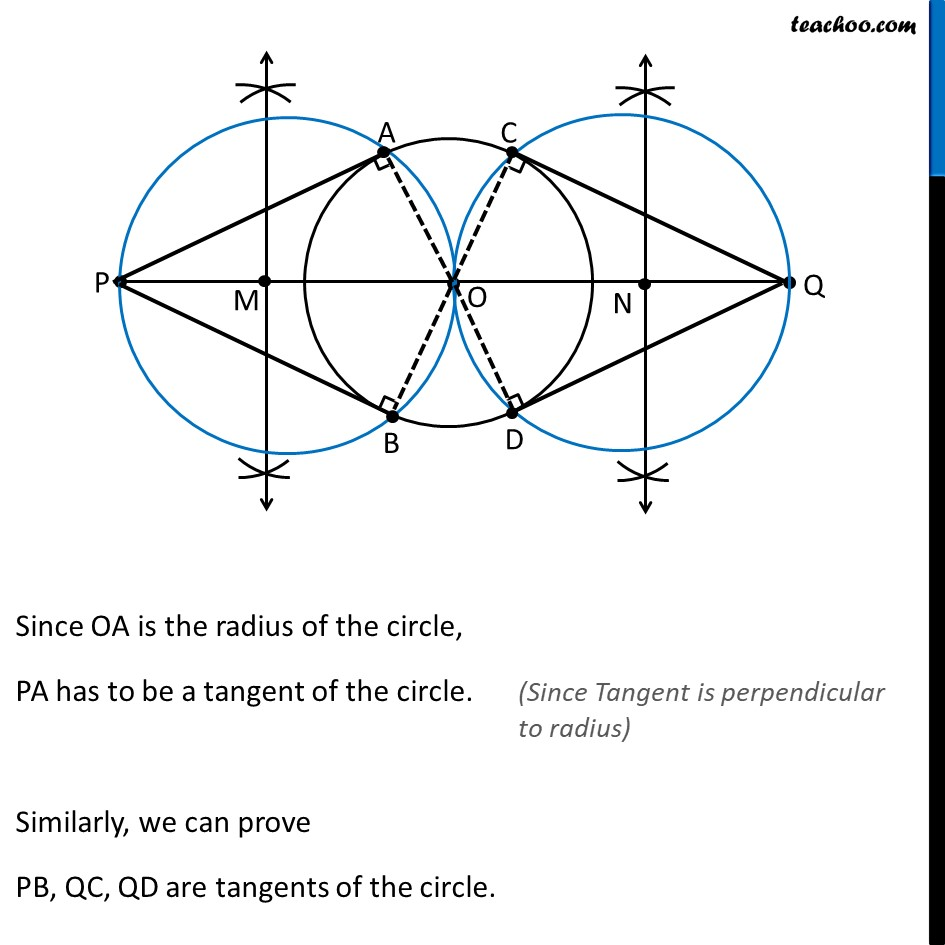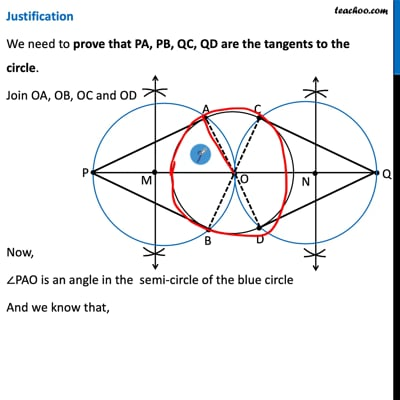This video is only available for Teachoo black users

Maths Crash Course - Live lectures + all videos + Real time Doubt solving!

### Transcript

Ex 11.2, 3 Draw a circle of radius 3 cm. Take two points P and Q on one of its extended diameter each at a distance of 7 cm from its centre. Draw tangents to the circle from these two points P and Q. Steps of construction Draw a circle of radius 3 cm Draw diameter of circle, and extend it and mark points P and Q, 7 cm from the center Let’s first draw tangent from point P 3. Make perpendicular bisector of PO Let M be the midpoint of PO. 4 Taking M as centre and MO as radius, draw a circle. 5. Let it intersect the given circle at points A and B. 6. Join PA and PB. Now, we draw tangent from point Q Similarly we draw tangent from point Q ∴ QC and QD are the tangents from point Q Justification We need to prove that PA, PB, QC, QD are the tangents to the circle. Join OA, OB, OC and OD Now, ∠PAO is an angle in the semi-circle of the blue circle And we know that, Angle in a semi-circle is a right angle. ∴ ∠PAO = 90° ⇒ OA ⊥ PA Since OA is the radius of the circle, PA has to be a tangent of the circle. Similarly, we can prove PB, QC, QD are tangents of the circle.